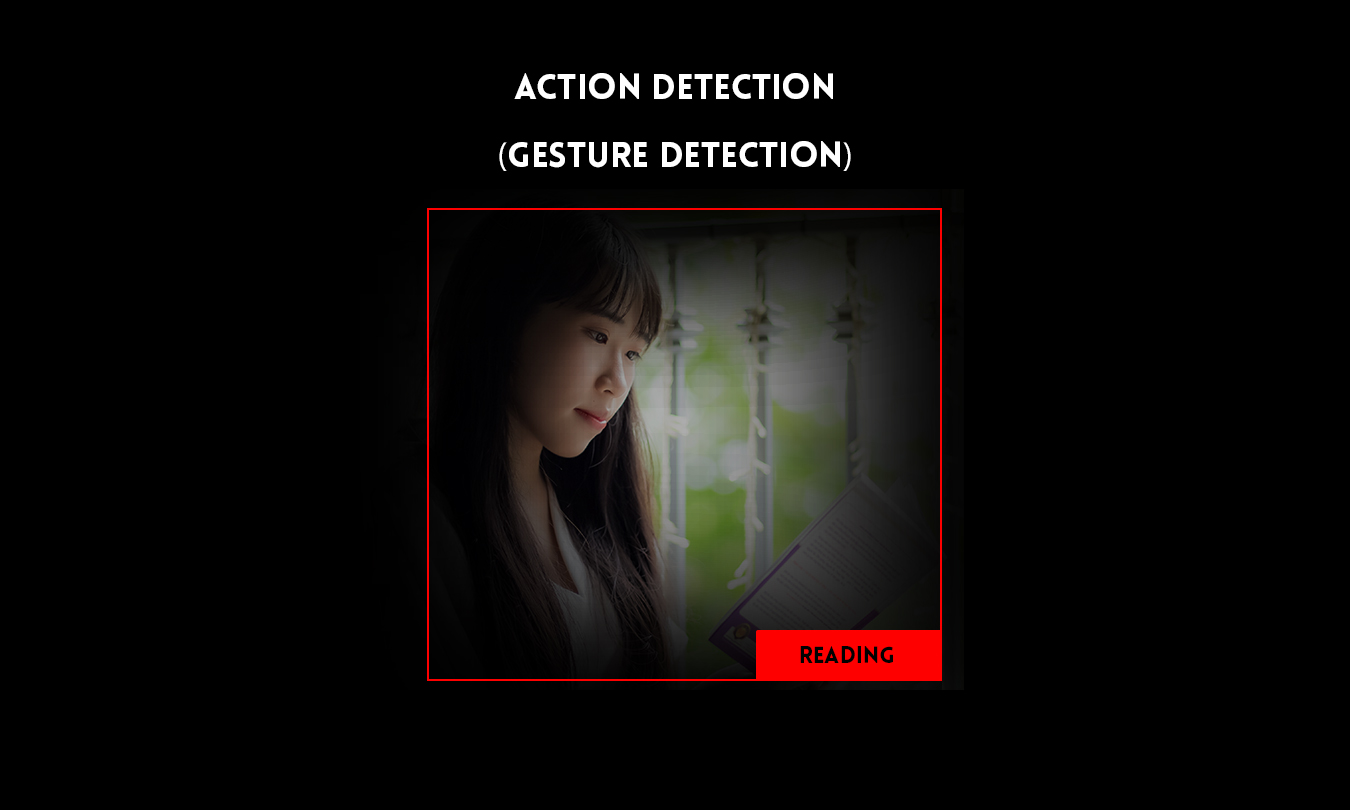## Action Detection (Gesture Detection)

Introduction
Action and gesture recognition has become very popular topics within the computer vision field in the last few years, especially after the application of deep learning in this domain. Similar to other areas of computer vision, the recent work on action and gesture recognition is mainly based on Convolutional Neural Networks (CNNs). In this blog, we show how to implement gesture detection and action detection using 3D Convolution Neural Network(CNN) without a bounding box. You can also do this project using OpenCV but there some issues in the background. Contour can’t easily detect hand so that a better idea is used 3D Convolution Neural Network(CNN).

Background
This project is to take video frame( 16 frame at time) as input, process the each 16 frame, train the machine learning algorithm or neural network to recognition hand movement and predict gesture or action every 16 frame.
This system is developed using OpenCV, keras and Tensorflow. Here, opencv is used for time prediction (webcam) and keras and tensorflow for training neural network algorithm.

Applications

• This project is aimed at developing software which will be helpful in Hand gesture recognition.
• In Advance filed we use Robot direction and identify human sign by a robot
• Also, we use in IoT for doing any task like when we show thumb up then fan(any device) automatically starts and when thumb down fan(any device) automatically stops

Requirements

• keras (pip install keras)
• tensorflow (pip install tensorflow)
• jester dataset (https://20bn.com/datasets/jester/)

Pre-requisite basic knowledge in concepts like

• basic knowledge of python and deep learning(Conv3D)
• basic knowledge of tensorflow framework
• basic knowledge of keras framework

Model Diagram
In Action Recognition and gesture recognition , we use two model one is 3D RESNET – 101 (figure 5.2 )and also manually create model that show figure 5.1Figure 5.1 : manually model (every 3D Convolution take 16 frame at time)Figure 5.2 : RESNET model architecture

How to use our pre-train model

2. Dataset label

3. Import dependency

• from tensorflow import keras
• import cv2
• import numpy as np
• import pandas as pd

`model = keras.models.load_model('PATH_PRETRAIN_MODEL /model.best.hdf5')`

`labels = pd.read_csv('PATH_OF_DATASET/jester-v1-labels.csv',header = None)`

6. Live predict

```buffer= []
video  = cv2.VideoCapture(0)
i = 1
cls = "Nothing"
while (vid.isOpened()):
if ret:
image = cv2.resize(frame,(96,64))
image = image/255.0
buffer.append(image)
if(i%16==0):
buffer = np.expand_dims(buffer,0)
cls = labels[np.argmax(model.predict(buffer))]
print(cls)
cv2.imshow('frame',frame)
buffer = []
i = i+1
cv2.putText(frame,cls,(5,30),cv2.FONT_HERSHEY_SIMPLEX, 1.0, (0, 0, 255),2)
cv2.imshow('frame',frame)
if cv2.waitKey(1) & 0xFF == ord('q'):
break
vid.release()
cv2.destroyAllWindows()```

Conclusion

• The purpose of this tutorial was to give you a brief idea about the 3D convolution and how to recognize action from video and implementing the real-time system. This project you also implement using Motion Fused Frames (MFFs) you find a reference from the internet also we give reference link below.
• As future work, we would like to analyze our approach on different modalities at more challenging tasks requiring human understanding in videos. We intend to find better ways to exploit the advantages of data-level fusion on CNNs for video analysis.

## Let's create the next BIG thing together

CREDITS